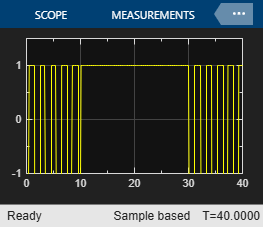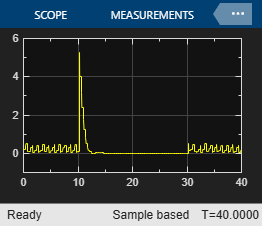## Switching Controllers Based on Optimal Costs

This example shows how to use the "optimal cost" outport of the MPC Controller block to switch between multiple model predictive controllers whose outputs are restricted to discrete values.

### Define Plant Model

The linear plant model is as follows::

```plant = ss(tf({1,1},{[1 1.2 1],[1 1]}),'min'); % Plant with 2 inputs and 1 output [A,B,C,D] = ssdata(plant); % Get state-space realization matrices, to be used in Simulink x0 = [0;0;0]; % Initial plant state ```

### Design MPC Controller

Specify input and output signal types.

```plant = setmpcsignals(plant,'MV',1,'MD',2); % First input is manipulated, second is measured disturbance ```

Design two MPC controllers with the MV constraints of `u=-1` and `u=1`, respectively. Only `u` at the current time is quantized. The subsequent calculated control actions may be any value between -1 and 1. The controller uses a receding horizon approach so these values don't actually go to the plants.

```Ts = 0.2; % Sampling time p = 20; % Prediction horizon m = 10; % Control horizon mpc1 = mpc(plant,Ts,p,m); % First MPC object mpc2 = mpc(plant,Ts,p,m); % Second MPC object % Specify weights mpc1.Weights = struct('MV',0,'MVRate',.3,'Output',1); % Weights mpc2.Weights = struct('MV',0,'MVRate',.3,'Output',1); % Weights % Specify constraints mpc1.MV = struct('Min',[-1;-1],'Max',[-1;1]); % Constraints on the manipulated variable: u = -1 mpc2.MV = struct('Min',[1;-1],'Max',[1;1]); % Constraints on the manipulated variable: u = 1 ```
```-->The "Weights.ManipulatedVariables" property of "mpc" object is empty. Assuming default 0.00000. -->The "Weights.ManipulatedVariablesRate" property of "mpc" object is empty. Assuming default 0.10000. -->The "Weights.OutputVariables" property of "mpc" object is empty. Assuming default 1.00000. -->The "Weights.ManipulatedVariables" property of "mpc" object is empty. Assuming default 0.00000. -->The "Weights.ManipulatedVariablesRate" property of "mpc" object is empty. Assuming default 0.10000. -->The "Weights.OutputVariables" property of "mpc" object is empty. Assuming default 1.00000. ```

To run this example, Simulink® is required.

```if ~mpcchecktoolboxinstalled('simulink') disp('Simulink(R) is required to run this example.') return end % Specify signals: Tstop = 40; ref.time = 0:Ts:(Tstop+p*Ts); ref.signals.values = double(ref.time>10)'; % Step change in reference signal at time t=10 md.time = ref.time; md.signals.values = double(md.time>30)'; % Step change in measured disturbance signal at time t=30 ```

Open and simulate the Simulink® model:

```mdl = 'mpc_optimalcost'; open_system(mdl); % Open Simulink(R) Model sim(mdl,Tstop); % Start Simulation ```
```-->Converting model to discrete time. -->Assuming output disturbance added to measured output channel #1 is integrated white noise. -->The "Model.Noise" property of the "mpc" object is empty. Assuming white noise on each measured output channel. -->Converting model to discrete time. -->Assuming output disturbance added to measured output channel #1 is integrated white noise. -->The "Model.Noise" property of the "mpc" object is empty. Assuming white noise on each measured output channel. ```Note that:

• From time 0 to time 10, the control action keeps switching between MPC1 (-1) and MPC2 (+1). This is because the reference signal is 0 and it requires a controller output at 0 to reach steady state, which cannot be achieved with either MPC controller.

• From time 10 to 30, MPC2 control output (+1) is chosen because the reference signal becomes +1 and it requires a controller output at +1 to reach steady state (plant gain is 1), which can be achieved by MPC2.

• From time 30 to 40, control action starts switching again. This is because with the presence of measured disturbance (+1), MPC1 leads to a steady state of 0 and MPC2 leads to a steady state of +2, while the reference signal still requires +1.

### Simulate Using MPCMOVE Command

Use `mpcmove` to perform step-by-step simulation and compute current MPC control action:

```[Ad,Bd,Cd,Dd] = ssdata(c2d(plant,Ts)); % Discrete-time dynamics Nsteps = round(Tstop/Ts); % Number of simulation steps ```

Initialize matrices for storing simulation results

```YY = zeros(Nsteps+1,1); RR = zeros(Nsteps+1,1); UU = zeros(Nsteps+1,1); COST = zeros(Nsteps+1,1); x = x0; % Initial plant state xt1 = mpcstate(mpc1); % Initial state of the MPC controller #1 xt2 = mpcstate(mpc2); % Initial state of the MPC controller #2 ```

Start simulation.

```for td=0:Nsteps % Construct signals v = md.signals.values(td+1); r = ref.signals.values(td+1); % Plant equations: output update y = Cd*x + Dd(:,2)*v; % Compute both MPC moves options = mpcmoveopt; options.OnlyComputeCost = true; % the last input argument tells "mpcmove" to only compute the optimal cost [u1,Info1] = mpcmove(mpc1,xt1,y,r,v,options); [u2,Info2] = mpcmove(mpc2,xt2,y,r,v,options); % Compare the resulting optimal costs and choose the input value % corresponding to the smallest cost if Info1.Cost<=Info2.Cost u = u1; cost = Info1.Cost; % Update internal MPC state to the correct value xt2.Plant = xt1.Plant; xt2.Disturbance = xt1.Disturbance; xt2.LastMove = xt1.LastMove; else u = u2; cost = Info2.Cost; % Update internal MPC state to the correct value xt1.Plant = xt2.Plant; xt1.Disturbance = xt2.Disturbance; xt1.LastMove = xt2.LastMove; end % Store plant information YY(td+1) = y; RR(td+1) = r; UU(td+1) = u; COST(td+1) = cost; % Plant equations: state update x = Ad*x + Bd(:,1)*u + Bd(:,2)*v; end ```

Plot the results of `mpcmove` to compare with the simulation results obtained in Simulink®:

```subplot(131) plot((0:Nsteps)*Ts,[YY,RR]); % Plot output and reference signals grid title('OV and Reference') subplot(132) plot((0:Nsteps)*Ts,UU); % Plot manipulated variable grid title('MV') subplot(133) plot((0:Nsteps)*Ts,COST); % Plot optimal MPC value function grid title('Optimal cost') ```These plots resemble the plots in the scopes in the Simulink® model.

```bdclose(mdl); ```